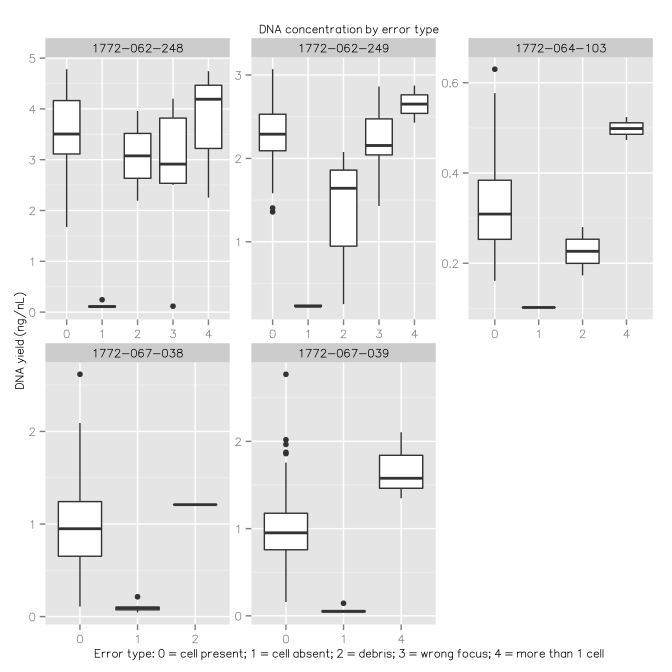# Combined analysis of Fucci fluorescence and cDNA concentration

## Summary

After visual inspection, chambers with no cells or with defect in the imaging were flagged for removal. The DNA yield for the same chambers was also very low. Conversely, in the absence of cells, the proportion of spikes was highest. This confirms the accuracy of the visual inspection and confirms that the conversion between C1 chip coordinates and 96-well plate coordinates was done correctly.

In the output file `combined.csv`, a column called `Discard` indicates if the cell fails any of the quality controls.

## Datasets

• The non corrected fluorescence values are produced as explained in Fluorescence-measured-in-ImageJ.html.
• The background and batch effect correction of the fluorescence values is explained in BackgroundCorrection.html
• The cDNA concentration values are produced as explained in cDNA_concentration.html.
• Normalised spike counts: `control-sequences/spikes.norm.csv`, see control-sequences.html.
• Location of the positive and negative controls: `controls.csv`, as indicated by the operators T. K., (email on Mon, 24 Mar 2014 20:06:36 +0900) and S. K. (e-mail on Tue, 25 Mar 2014 09:02:50 +0900).

## Quality control

``````library(gdata)   # for drop.levels()
library(ggplot2) # for the plots
library(scales)  # for trans_new()
``````

### Load and merge the fluorescence and concentration values

The `qc` table is assembled by merging multiple data sources. It is then saved as `qc.full`. In the steps that follow, entries that do not pass quality controls will be removed from the `qc` table.

``````fl <- read.csv("../fluorescence/Results_fluorescence.csv")
fl\$Error <- factor(fl\$Error)
fl <- fl[,c(1,28,30,31,32)]

correctedFl <- correctedFl[,1:3]

qc <- merge(fl, correctedFl[,1:3], all=TRUE)

# pg as short name for picogreen
pg\$Column <- factor(pg\$Column)
pg\$cell_id <- paste(pg\$Run, pg\$Well, sep='_')
qc <- merge(pg, qc, by=c('cell_id', 'Run', 'Well'), all=TRUE)

qc <- merge(qc, spikes, all=TRUE)

summary(controls)
``````
``````##            Run         Well   Control
##  1772-062-248:2   A02    :2   NC:5
##  1772-062-249:2   A10    :1   PC:5
##  1772-064-103:2   B01    :1
##  1772-067-038:2   C09    :1
##  1772-067-039:2   F08    :1
##                   F12    :1
##                   (Other):3
``````
``````controls\$cell_id <- paste(controls\$Run, controls\$Well, sep='_')
qc <- merge(qc, controls, by=c('cell_id', 'Run', 'Well'), all=TRUE)
rownames(qc) <- qc\$cell_id

hiseq <- hiseq[,c(1,10, 15:17,19,20)]
qc <- merge(qc, hiseq, by=c('cell_id', 'Run', 'Well', 'Row', 'Column'))

# replace error type with numbers
error <- sapply(strsplit(as.character(qc\$Error),"-", fixed = TRUE),"[[", 1)
qc\$Error <- error

qc.full <- qc
``````

### Remove the samples that were replaced by positive or negative controls.

``````qc <- subset(qc, is.na(qc\$Control))
``````

### Visual curation

Visual curation of the fluorescence pictures (`Error` field, see Fluorescence-measured-in-ImageJ.html) eliminated the chambers where it was not sure wether a healthy single cell was captured, in good concordance with the DNA yields. In the absence of a cell the libraries are mostly made of spikes.

### Remove the cells for which there are no image files.

``````qc <- subset(qc, !is.na(qc\$Error))
``````
``````qplot(data = qc, Error, mean_ch2 + mean_ch3, geom = "boxplot"
) + facet_wrap(~Run, scales = "free") + ggtitle('Uncorrected fluorescence by error type') + scale_x_discrete('Error type: 0 = cell present; 1 = cell absent; 2 = debris; 3 = wrong focus; 4 = more than 1 cell')
``````
``````## Error in eval(expr, envir, enclos): object 'mean_ch2' not found
``````

#### DNA concentration.

``````qplot(data = qc, Error, Concentration, geom = "boxplot"
) + facet_wrap(~Run, scales = "free") + ggtitle('DNA concentration by error type') + scale_x_discrete('Error type: 0 = cell present; 1 = cell absent; 2 = debris; 3 = wrong focus; 4 = more than 1 cell') + scale_y_continuous('DNA yield (ng/nL)')
``````Back to top

#### 18S rRNA.

``````qplot(data = qc, Error, rRNA_18S, geom = "boxplot"
) + facet_wrap(~Run, scales = "free") + ggtitle('18S rRNA by error type') + scale_x_discrete('Error type: 0 = cell present; 1 = cell absent; 2 = debris; 3 = wrong focus; 4 = more than 1 cell') + scale_y_continuous('rRNA 18S (CPM)')
``````
``````## Warning: Removed 5 rows containing non-finite values (stat_boxplot).
``````